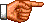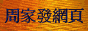# 點算的奧秘：含不動點或繼續點的排列問題

《點算的奧秘：廣義容斥原理》中，筆者推導了「廣義容斥原理」的公 式。在本節中，筆者將把這些公式應用於兩類特殊的排列問題。這些排列問題可以視為具有某些特殊限制的排 列問題，這些特殊限制有兩種：分別為「不動點」和「繼續點」。

(n − s)!    (1)

N(n, s) = C(n, s) Σ0 ≤ r ≤ n−s(−1)r C(n − s, r) nr+s    (2)

F(n, s) = C(n, s) Σ0 ≤ r ≤ n−s(−1)r C(n − s, r) (n − r − s)!    (3)

 C(n, s) × C(n − s, r) × (n − r − s)!
 n! (n − s)! = ----------------- × ---------------------- × (n − r − s)! (n − s)! × s! (n − s − r)! × r! n! = -------------- s! × r!

F(n, s) = (n! / s!) Σ0 ≤ r ≤ n−s(−1)r / r!     (4)

F(n, 0) = n! Σ0 ≤ r ≤ n(−1)r / r!    (5)

 F(5, 0) = 5! Σ0 ≤ r ≤ 5(−1)r / r! = 120 × (1/1 − 1/1 + 1/2 − 1/6 + 1/24 − 1/120) = 44 □

(n − s)!    (6)

S(n, s) = C(n − 1, s) Σ0 ≤ r ≤ n−1−s (−1)r C(n − 1 − s, r) (n − r − s)!    (7)

 C(n − 1, s) × C(n − 1 − s, r) × (n − r − s)!
 (n − 1)! (n − 1 − s)! = -------------------- × ------------------------- × (n − r − s)! (n − 1 − s)! × s! (n − 1 − s − r)! × r! (n − 1)! × (n − r − s) = ---------------------------- s! × r!

S(n, s) = ((n − 1)! / s!) Σ0 ≤ r ≤ n−1−s (−1)r (n − r − s) / r!    (8)

S(n, 0) = (n − 1)! Σ0 ≤ r ≤ n−1(−1)r (n − r) / r!    (9)

 S(5, 0) = 4! Σ0 ≤ r ≤ 4(−1)r (5 − r) / r! = 24 × (5/1 − 4/1 + 3/2 − 2/6 + 1/24) = 53 □<script type="text/javascript">(function (d, w) {var x = d.getElementsByTagName('SCRIPT');var f = function () {var s = d.createElement('SCRIPT');s.type = 'text/javascript';s.async = true;s.src = "//np.lexity.com/embed/YW/be0aa169de7f441c6473361be62c9ef6?id=ddad453e7753";x.parentNode.insertBefore(s, x);};w.attachEvent ? w.attachEvent('onload',f) :w.addEventListener('load',f,false);}(document, window));</script>